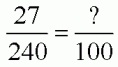SEARCH HOMEMath Central Quandaries & QueriesQuestion from Keshia, a student: If there are 27 grams of sugar in 240 mililiters, How many grams are in 100 mililiters? I have been trying to figure this question out for about an hour and I cannot seem to get it. Thank you for your help.Hi Keshia,

First a question about your question, from Chris:

Unless I'm terribly confused, which is likely, the numbers are off by a factor of 10 -- I thought a gram and a milliliter (note the two l's) were about the same thing (for sugar) -- I'd believe 270 grams of sugar in 240 milliliters.  Please check it out before answering the question.

Chris
--

If your numbers are correct, then maybe you are talking about the number of grams of sugar dissolved in a solution? If this is the case then it might make sense to have 27 grams of sugar in 240 milliliters (of the solution). If you then take just 100ml of that solution, then the ratio of sugar to solution should not change (WHY? Does this seem reasonable to you?) So you can set up a relation: 27 : 240 = ? : 100 . Or,Does this help?

-HaleyMath Central is supported by the University of Regina and The Pacific Institute for the Mathematical Sciences.